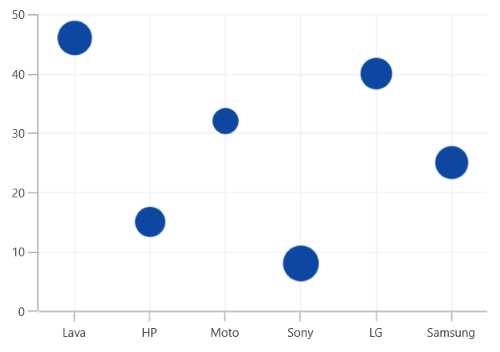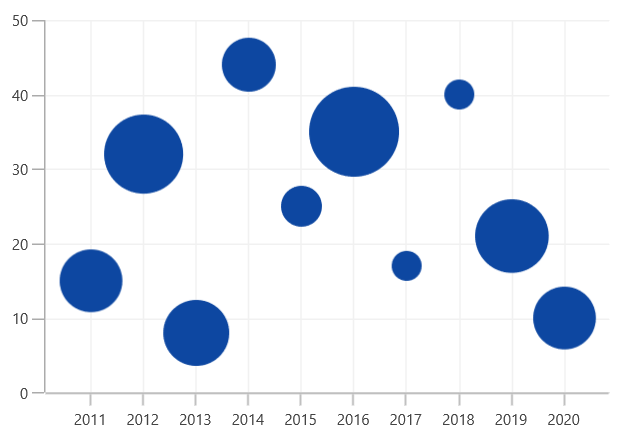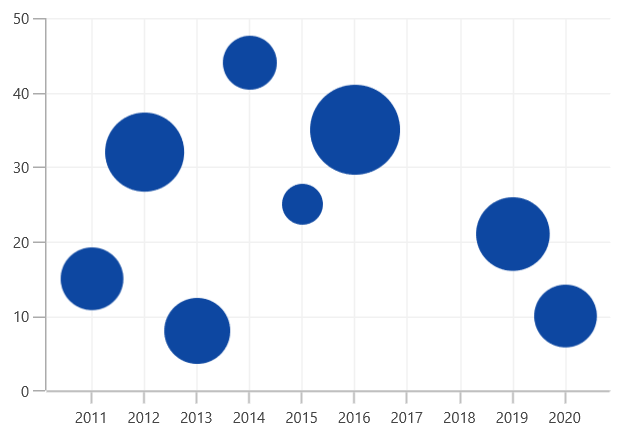# Bubble Chart in WinUI Chart (SfCartesianChart)

11 Oct 20214 minutes to read

## Bubble Chart

The BubbleSeries is represented by closely packed circles, whose areas are proportional to the quantities. The size of the bubble series is relative proportional to the value bind with the series using the Size property. You can set the constraints on this size using the MinimumRadius and MaximumRadius properties.

To render a bubble series, create an instance of BubbleSeries and add to the Series collection property of SfCartesianChart as shown in the following code.

``````<chart:SfCartesianChart>

<chart:SfCartesianChart.PrimaryAxis>
<chart:CategoryAxis />
</chart:SfCartesianChart.PrimaryAxis>

<chart:SfCartesianChart.SecondaryAxis>
<chart:NumericalAxis />
</chart:SfCartesianChart.SecondaryAxis>

...
<chart:SfCartesianChart>``````
``````SfCartesianChart chart = new SfCartesianChart();
CategoryAxis primaryAxis = new CategoryAxis();
chart.PrimaryAxis = primaryAxis;
NumericalAxis secondaryAxis = new NumericalAxis();
chart.SecondaryAxis = secondaryAxis;
. . .
BubbleSeries series = new BubbleSeries()
{
ItemsSource = new ViewModel().Data,
XBindingPath = "XValue",
YBindingPath = "YValue",
Size = "Size",
};

...``````## Show zero size bubbles

The zero size bubble segments can be enabled or disabled by using the ShowZeroBubbles property. The default value of this property is `True`.

``````<chart:SfCartesianChart>

<chart:SfCartesianChart.PrimaryAxis>
<chart:CategoryAxis />
</chart:SfCartesianChart.PrimaryAxis>

<chart:SfCartesianChart.SecondaryAxis>
<chart:NumericalAxis />
</chart:SfCartesianChart.SecondaryAxis>

<chart:BubbleSeries ItemsSource="{Binding Data}" XBindingPath="XValue" YBindingPath="YValue" Size="Size" ShowZeroBubbles="True"/>
...
<chart:SfCartesianChart>``````
``````SfCartesianChart chart = new SfCartesianChart();
CategoryAxis primaryAxis = new CategoryAxis();
chart.PrimaryAxis = primaryAxis;
NumericalAxis secondaryAxis = new NumericalAxis();
chart.SecondaryAxis = secondaryAxis;
. . .
BubbleSeries series = new BubbleSeries()
{
ItemsSource = new ViewModel().Data,
XBindingPath = "XValue",
YBindingPath = "YValue",
Size = "Size",
ShowZeroBubbles = true,
};

...````````````<chart:SfCartesianChart>

<chart:SfCartesianChart.PrimaryAxis>
<chart:CategoryAxis />
</chart:SfCartesianChart.PrimaryAxis>

<chart:SfCartesianChart.SecondaryAxis>
<chart:NumericalAxis />
</chart:SfCartesianChart.SecondaryAxis>

<chart:BubbleSeries ItemsSource="{Binding Data}" XBindingPath="XValue"
YBindingPath="YValue" Size="Size"
ShowZeroBubbles="False"/>
...
<chart:SfCartesianChart>``````
``````SfCartesianChart chart = new SfCartesianChart();
CategoryAxis primaryAxis = new CategoryAxis();
chart.PrimaryAxis = primaryAxis;
NumericalAxis secondaryAxis = new NumericalAxis();
chart.SecondaryAxis = secondaryAxis;
. . .
BubbleSeries series = new BubbleSeries()
{
ItemsSource = new ViewModel().Data,
XBindingPath = "XValue",
YBindingPath = "YValue",
Size = "Size",
ShowZeroBubbles = false,
};